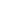# Fitness: round holes and square holes, pegged

Wolfram Mathworld bores down into a much-discussed-by-others-but-usually-idly question about pegs and holes:

The answer to the question “which fits better, a round peg in a square hole, or a square peg in a round hole?” can be interpreted as asking which is larger, the ratio of the area of a circle to its circumscribed square, or the area of thesquare to its circumscribed circle? In two dimensions, the ratios areand, respectively. Therefore, a round peg fits better into a square hole than a square peg fits into a round hole (Wells 1986, p. 74).However…

REFERENCES:

Singmaster, D. “On Round Pegs in Square Holes and Square Pegs in Round Holes.” Math. Mag. 37, 335-339, 1964.

Sloane, N. J. A. Sequence A127454 in “The On-Line Encyclopedia of Integer Sequences.”

Wells, D. The Penguin Dictionary of Curious and Interesting Numbers. Middlesex, England: Penguin Books, p. 74, 1986.

[via Cliff Pickover]